# RCD-Lecture 6-4.pdf

May. 27, 2023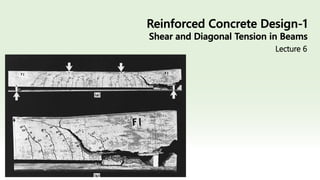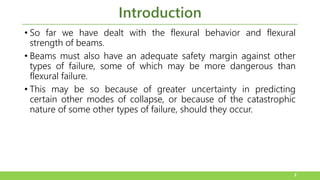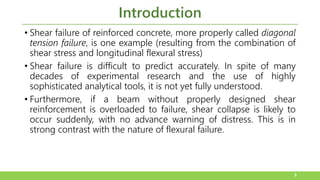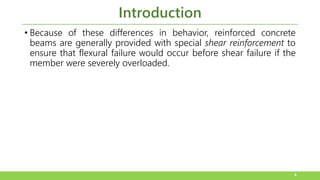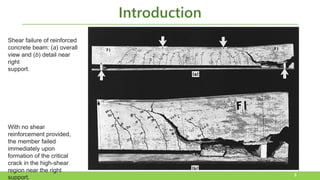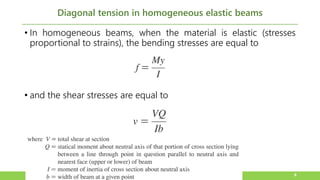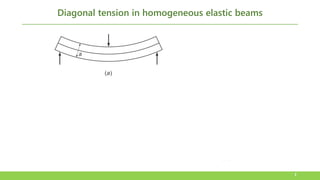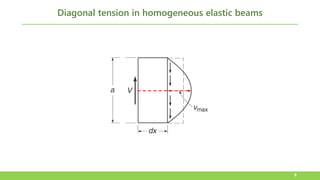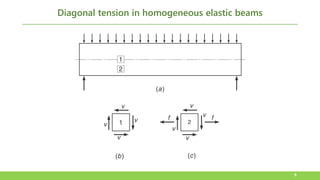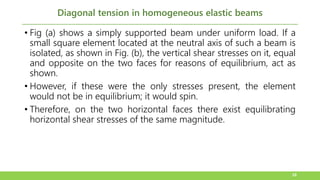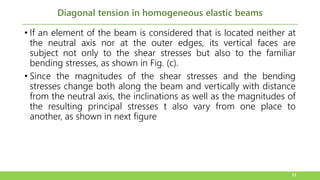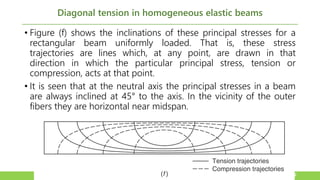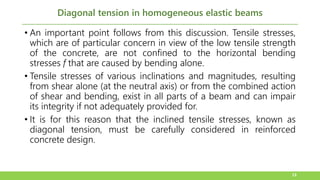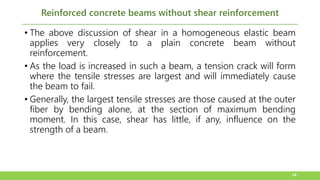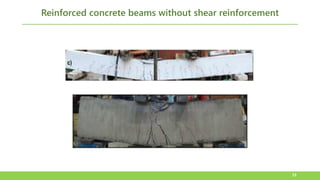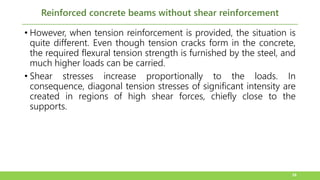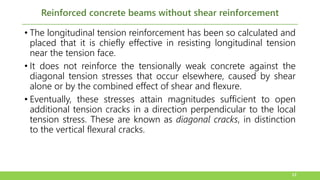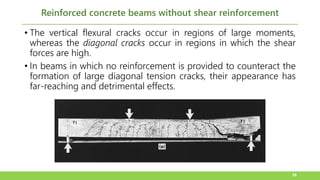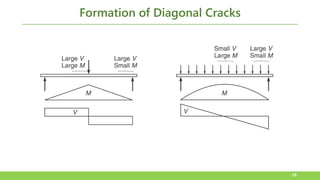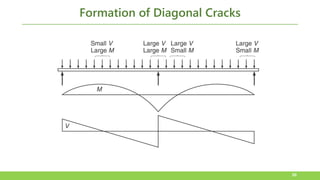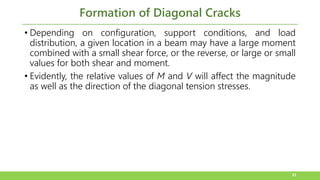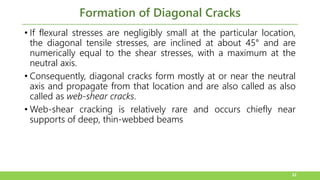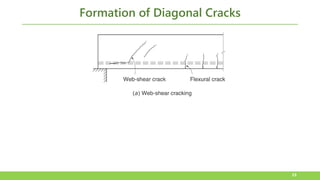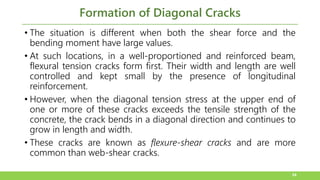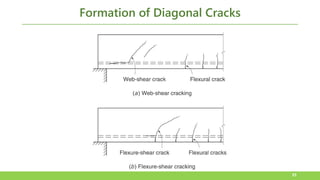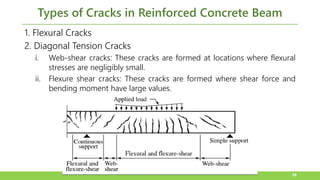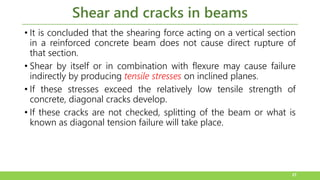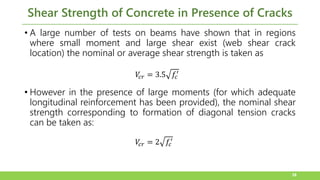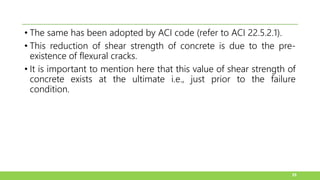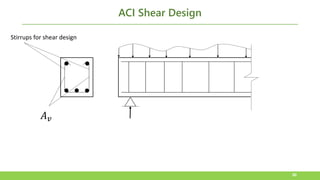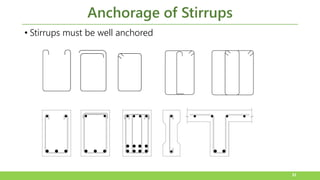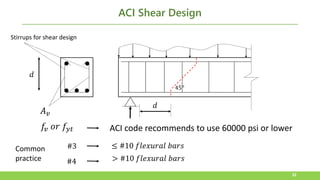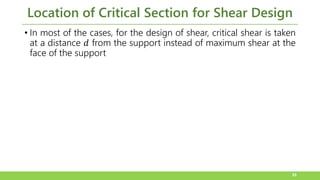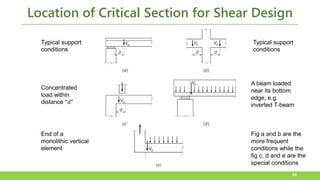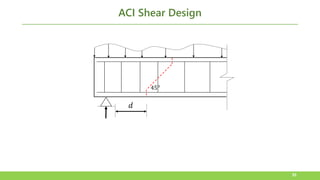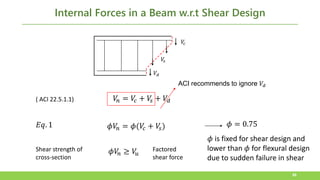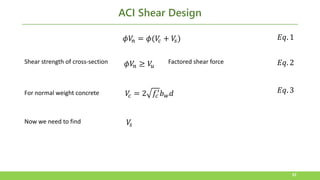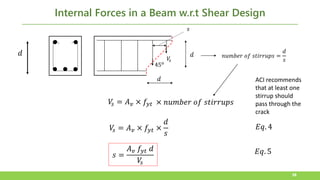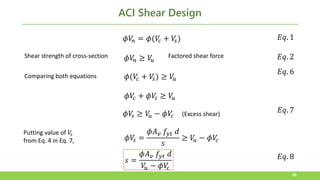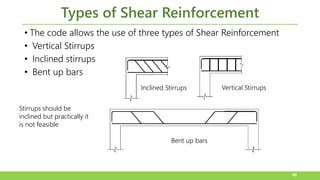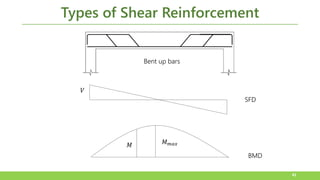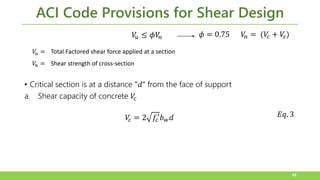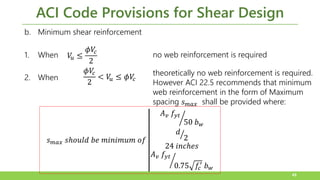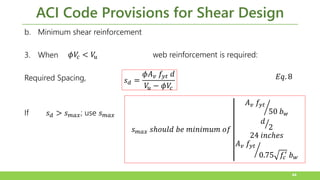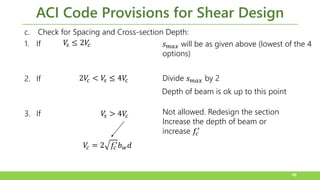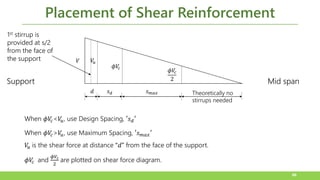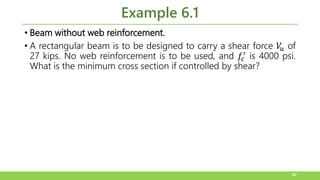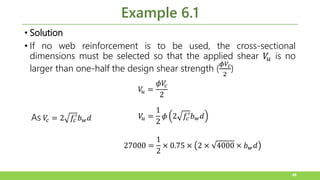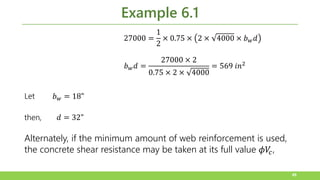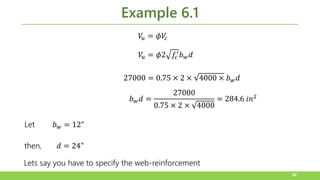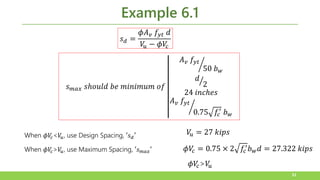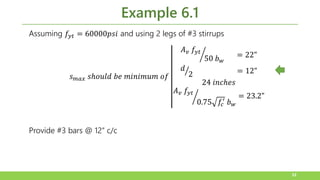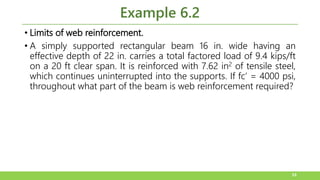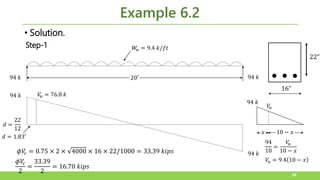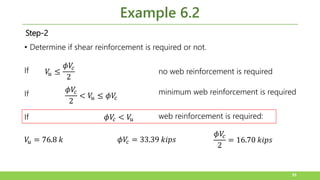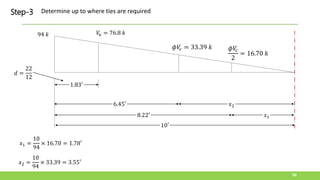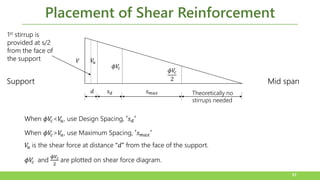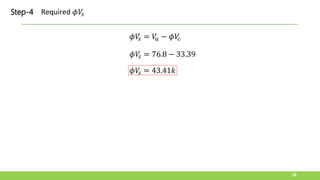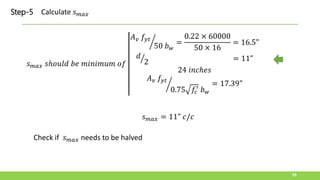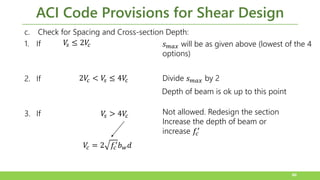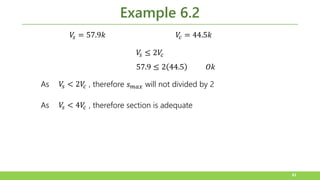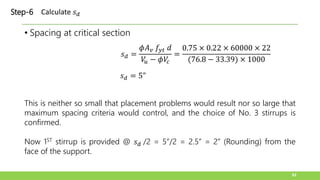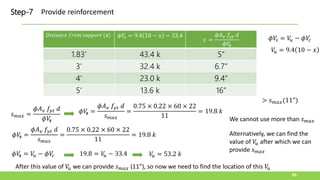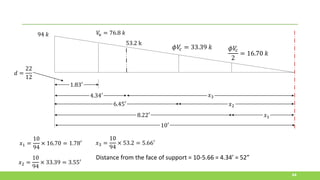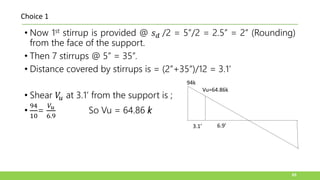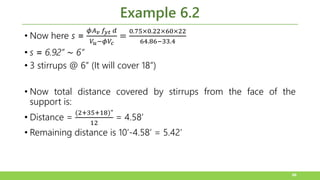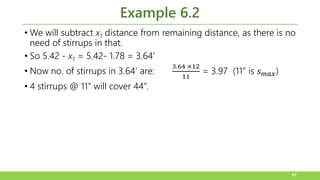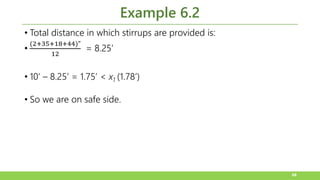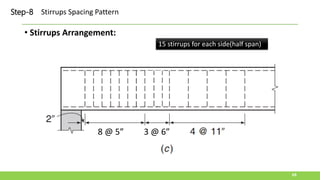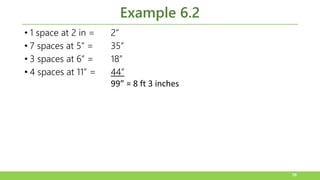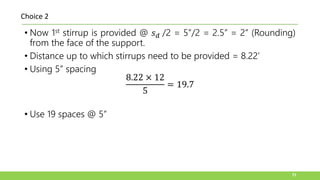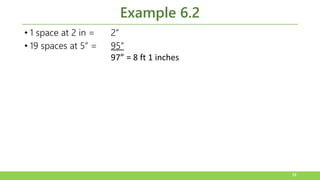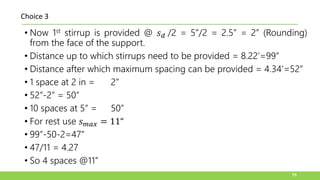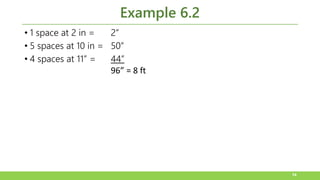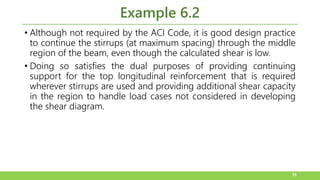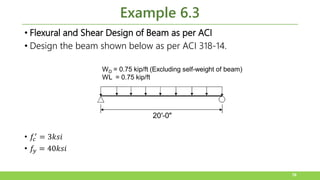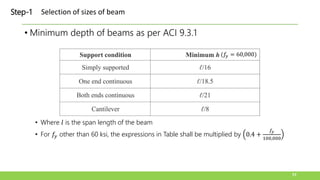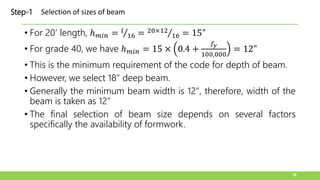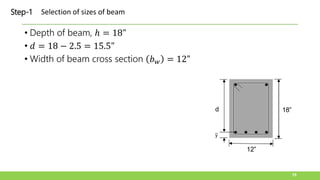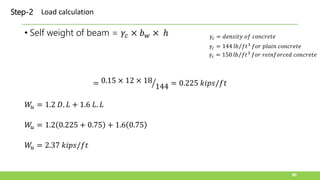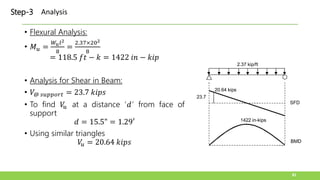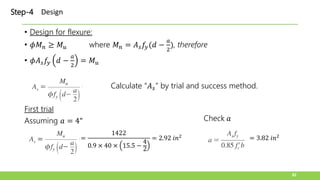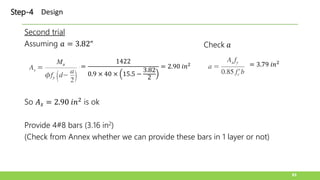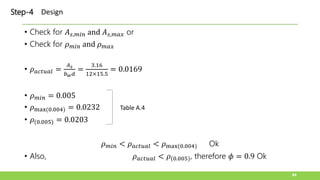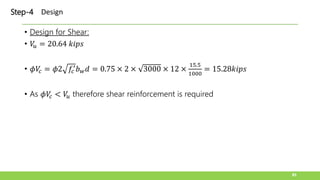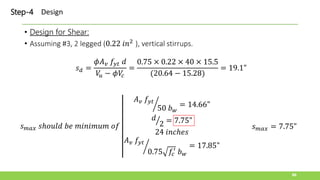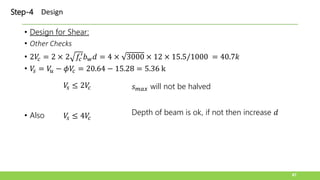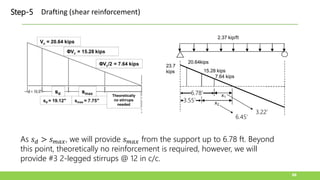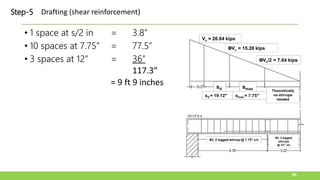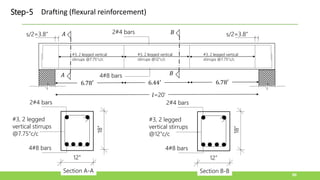1 of 90

### RCD-Lecture 6-4.pdf

• 2. Introduction • So far we have dealt with the flexural behavior and flexural strength of beams. • Beams must also have an adequate safety margin against other types of failure, some of which may be more dangerous than flexural failure. • This may be so because of greater uncertainty in predicting certain other modes of collapse, or because of the catastrophic nature of some other types of failure, should they occur. 2
• 3. Introduction • Shear failure of reinforced concrete, more properly called diagonal tension failure, is one example (resulting from the combination of shear stress and longitudinal flexural stress) • Shear failure is difficult to predict accurately. In spite of many decades of experimental research and the use of highly sophisticated analytical tools, it is not yet fully understood. • Furthermore, if a beam without properly designed shear reinforcement is overloaded to failure, shear collapse is likely to occur suddenly, with no advance warning of distress. This is in strong contrast with the nature of flexural failure. 3
• 4. Introduction • Because of these differences in behavior, reinforced concrete beams are generally provided with special shear reinforcement to ensure that flexural failure would occur before shear failure if the member were severely overloaded. 4
• 5. Introduction 5 With no shear reinforcement provided, the member failed immediately upon formation of the critical crack in the high-shear region near the right support. Shear failure of reinforced concrete beam: (a) overall view and (b) detail near right support.
• 6. Diagonal tension in homogeneous elastic beams • In homogeneous beams, when the material is elastic (stresses proportional to strains), the bending stresses are equal to • and the shear stresses are equal to 6
• 7. Diagonal tension in homogeneous elastic beams 7
• 8. Diagonal tension in homogeneous elastic beams 8
• 9. Diagonal tension in homogeneous elastic beams 9
• 10. Diagonal tension in homogeneous elastic beams 10 • Fig (a) shows a simply supported beam under uniform load. If a small square element located at the neutral axis of such a beam is isolated, as shown in Fig. (b), the vertical shear stresses on it, equal and opposite on the two faces for reasons of equilibrium, act as shown. • However, if these were the only stresses present, the element would not be in equilibrium; it would spin. • Therefore, on the two horizontal faces there exist equilibrating horizontal shear stresses of the same magnitude.
• 11. Diagonal tension in homogeneous elastic beams 11 • If an element of the beam is considered that is located neither at the neutral axis nor at the outer edges, its vertical faces are subject not only to the shear stresses but also to the familiar bending stresses, as shown in Fig. (c). • Since the magnitudes of the shear stresses and the bending stresses change both along the beam and vertically with distance from the neutral axis, the inclinations as well as the magnitudes of the resulting principal stresses t also vary from one place to another, as shown in next figure
• 12. Diagonal tension in homogeneous elastic beams 12 • Figure (f) shows the inclinations of these principal stresses for a rectangular beam uniformly loaded. That is, these stress trajectories are lines which, at any point, are drawn in that direction in which the particular principal stress, tension or compression, acts at that point. • It is seen that at the neutral axis the principal stresses in a beam are always inclined at 45° to the axis. In the vicinity of the outer fibers they are horizontal near midspan.
• 13. Diagonal tension in homogeneous elastic beams 13 • An important point follows from this discussion. Tensile stresses, which are of particular concern in view of the low tensile strength of the concrete, are not confined to the horizontal bending stresses f that are caused by bending alone. • Tensile stresses of various inclinations and magnitudes, resulting from shear alone (at the neutral axis) or from the combined action of shear and bending, exist in all parts of a beam and can impair its integrity if not adequately provided for. • It is for this reason that the inclined tensile stresses, known as diagonal tension, must be carefully considered in reinforced concrete design.
• 14. Reinforced concrete beams without shear reinforcement 14 • The above discussion of shear in a homogeneous elastic beam applies very closely to a plain concrete beam without reinforcement. • As the load is increased in such a beam, a tension crack will form where the tensile stresses are largest and will immediately cause the beam to fail. • Generally, the largest tensile stresses are those caused at the outer fiber by bending alone, at the section of maximum bending moment. In this case, shear has little, if any, influence on the strength of a beam.
• 15. Reinforced concrete beams without shear reinforcement 15
• 16. Reinforced concrete beams without shear reinforcement 16 • However, when tension reinforcement is provided, the situation is quite different. Even though tension cracks form in the concrete, the required flexural tension strength is furnished by the steel, and much higher loads can be carried. • Shear stresses increase proportionally to the loads. In consequence, diagonal tension stresses of significant intensity are created in regions of high shear forces, chiefly close to the supports.
• 17. Reinforced concrete beams without shear reinforcement 17 • The longitudinal tension reinforcement has been so calculated and placed that it is chiefly effective in resisting longitudinal tension near the tension face. • It does not reinforce the tensionally weak concrete against the diagonal tension stresses that occur elsewhere, caused by shear alone or by the combined effect of shear and flexure. • Eventually, these stresses attain magnitudes sufficient to open additional tension cracks in a direction perpendicular to the local tension stress. These are known as diagonal cracks, in distinction to the vertical flexural cracks.
• 18. Reinforced concrete beams without shear reinforcement 18 • The vertical flexural cracks occur in regions of large moments, whereas the diagonal cracks occur in regions in which the shear forces are high. • In beams in which no reinforcement is provided to counteract the formation of large diagonal tension cracks, their appearance has far-reaching and detrimental effects.
• 21. Formation of Diagonal Cracks 21 • Depending on configuration, support conditions, and load distribution, a given location in a beam may have a large moment combined with a small shear force, or the reverse, or large or small values for both shear and moment. • Evidently, the relative values of M and V will affect the magnitude as well as the direction of the diagonal tension stresses.
• 22. Formation of Diagonal Cracks 22 • If flexural stresses are negligibly small at the particular location, the diagonal tensile stresses, are inclined at about 45° and are numerically equal to the shear stresses, with a maximum at the neutral axis. • Consequently, diagonal cracks form mostly at or near the neutral axis and propagate from that location and are also called as also called as web-shear cracks. • Web-shear cracking is relatively rare and occurs chiefly near supports of deep, thin-webbed beams
• 24. Formation of Diagonal Cracks 24 • The situation is different when both the shear force and the bending moment have large values. • At such locations, in a well-proportioned and reinforced beam, flexural tension cracks form first. Their width and length are well controlled and kept small by the presence of longitudinal reinforcement. • However, when the diagonal tension stress at the upper end of one or more of these cracks exceeds the tensile strength of the concrete, the crack bends in a diagonal direction and continues to grow in length and width. • These cracks are known as flexure-shear cracks and are more common than web-shear cracks.
• 26. Types of Cracks in Reinforced Concrete Beam 1. Flexural Cracks 2. Diagonal Tension Cracks i. Web-shear cracks: These cracks are formed at locations where flexural stresses are negligibly small. ii. Flexure shear cracks: These cracks are formed where shear force and bending moment have large values. 26
• 27. Shear and cracks in beams • It is concluded that the shearing force acting on a vertical section in a reinforced concrete beam does not cause direct rupture of that section. • Shear by itself or in combination with flexure may cause failure indirectly by producing tensile stresses on inclined planes. • If these stresses exceed the relatively low tensile strength of concrete, diagonal cracks develop. • If these cracks are not checked, splitting of the beam or what is known as diagonal tension failure will take place. 27
• 28. Shear Strength of Concrete in Presence of Cracks • A large number of tests on beams have shown that in regions where small moment and large shear exist (web shear crack location) the nominal or average shear strength is taken as • However in the presence of large moments (for which adequate longitudinal reinforcement has been provided), the nominal shear strength corresponding to formation of diagonal tension cracks can be taken as: 28 𝑉 𝑐𝑟 = 3.5 𝑓𝑐 ′ 𝑉 𝑐𝑟 = 2 𝑓𝑐 ′
• 29. • The same has been adopted by ACI code (refer to ACI 22.5.2.1). • This reduction of shear strength of concrete is due to the pre- existence of flexural cracks. • It is important to mention here that this value of shear strength of concrete exists at the ultimate i.e., just prior to the failure condition. 29
• 30. ACI Shear Design 30 Stirrups for shear design 𝐴𝑣
• 31. Anchorage of Stirrups • Stirrups must be well anchored 31
• 32. ACI Shear Design 32 Stirrups for shear design 𝐴𝑣 𝑓𝑣 𝑜𝑟 𝑓𝑦𝑡 ACI code recommends to use 60000 psi or lower #3 #4 ≤ #10 𝑓𝑙𝑒𝑥𝑢𝑟𝑎𝑙 𝑏𝑎𝑟𝑠 > #10 𝑓𝑙𝑒𝑥𝑢𝑟𝑎𝑙 𝑏𝑎𝑟𝑠 Common practice 𝑑 45𝑜 𝑑
• 33. Location of Critical Section for Shear Design • In most of the cases, for the design of shear, critical shear is taken at a distance 𝑑 from the support instead of maximum shear at the face of the support 33
• 34. Location of Critical Section for Shear Design 34 Typical support conditions Typical support conditions Concentrated load within distance “𝑑” A beam loaded near its bottom edge, e.g. inverted T-beam End of a monolithic vertical element Fig a and b are the more frequent conditions while the fig c, d and e are the special conditions
• 36. Internal Forces in a Beam w.r.t Shear Design 36 𝑉 𝑐 𝑉𝑑 𝑉 𝑠 𝑉 𝑛 = 𝑉 𝑐 + 𝑉 𝑠 + 𝑉𝑑 ACI recommends to ignore 𝑉𝑑 𝜙𝑉 𝑛 = 𝜙(𝑉 𝑐 + 𝑉 𝑠) 𝜙 = 0.75 𝜙 is fixed for shear design and lower than 𝜙 for flexural design due to sudden failure in shear 𝜙𝑉 𝑛 ≥ 𝑉 𝑢 Shear strength of cross-section Factored shear force 𝐸𝑞. 1 ( ACI 22.5.1.1)
• 37. ACI Shear Design 37 𝜙𝑉 𝑛 = 𝜙(𝑉 𝑐 + 𝑉 𝑠) 𝜙𝑉 𝑛 ≥ 𝑉 𝑢 Shear strength of cross-section Factored shear force 𝐸𝑞. 1 𝐸𝑞. 2 𝑉 𝑐 = 2 𝑓𝑐 ′ 𝑏𝑤𝑑 For normal weight concrete 𝐸𝑞. 3 𝑉 𝑠 Now we need to find
• 38. Internal Forces in a Beam w.r.t Shear Design 38 𝑉 𝑠 𝑑 45𝑜 𝑉 𝑠 = 𝐴𝑣 × 𝑓𝑦𝑡 𝑠 𝑑 𝑑 × 𝑛𝑢𝑚𝑏𝑒𝑟 𝑜𝑓 𝑠𝑡𝑖𝑟𝑟𝑢𝑝𝑠 𝑛𝑢𝑚𝑏𝑒𝑟 𝑜𝑓 𝑠𝑡𝑖𝑟𝑟𝑢𝑝𝑠 = 𝑑 𝑠 𝑉 𝑠 = 𝐴𝑣 × 𝑓𝑦𝑡 × 𝑑 𝑠 ACI recommends that at least one stirrup should pass through the crack 𝐸𝑞. 4 𝑠 = 𝐴𝑣 𝑓𝑦𝑡 𝑑 𝑉 𝑠 𝐸𝑞. 5
• 39. ACI Shear Design 39 𝜙𝑉 𝑛 = 𝜙(𝑉 𝑐 + 𝑉 𝑠) 𝜙𝑉 𝑛 ≥ 𝑉 𝑢 Shear strength of cross-section Factored shear force 𝐸𝑞. 1 𝐸𝑞. 2 Comparing both equations 𝜙(𝑉 𝑐 + 𝑉 𝑠) ≥ 𝑉 𝑢 𝐸𝑞. 6 𝜙𝑉 𝑐 + 𝜙𝑉 𝑠 ≥ 𝑉 𝑢 𝜙𝑉 𝑠 ≥ 𝑉 𝑢 − 𝜙𝑉 𝑐 𝐸𝑞. 7 Putting value of 𝑉 𝑠 from Eq. 4 in Eq. 7, (Excess shear) 𝜙𝑉 𝑠 = 𝜙𝐴𝑣 𝑓𝑦𝑡 𝑑 𝑠 ≥ 𝑉 𝑢 − 𝜙𝑉 𝑐 𝑠 = 𝜙𝐴𝑣 𝑓𝑦𝑡 𝑑 𝑉 𝑢 − 𝜙𝑉 𝑐 𝐸𝑞. 8
• 40. Types of Shear Reinforcement • The code allows the use of three types of Shear Reinforcement • Vertical Stirrups • Inclined stirrups • Bent up bars 40 Vertical Stirrups Inclined Stirrups Bent up bars Stirrups should be inclined but practically it is not feasible
• 41. Types of Shear Reinforcement 41 Bent up bars SFD BMD 𝑉 𝑀 𝑀𝑚𝑎𝑥
• 42. ACI Code Provisions for Shear Design • Critical section is at a distance "𝑑“ from the face of support a. Shear capacity of concrete 𝑉 𝑐 42 𝑉 𝑢 ≤ 𝜙𝑉 𝑛 𝜙 = 0.75 𝑉 𝑛 = (𝑉 𝑐 + 𝑉 𝑠) Shear strength of cross-section Total Factored shear force applied at a section 𝑉 𝑢 = 𝑉 𝑛 = 𝑉 𝑐 = 2 𝑓𝑐 ′ 𝑏𝑤𝑑 𝐸𝑞. 3
• 43. ACI Code Provisions for Shear Design b. Minimum shear reinforcement 1. When 2. When 43 𝑉 𝑢 ≤ 𝜙𝑉 𝑐 2 𝜙𝑉 𝑐 2 < 𝑉 𝑢 ≤ 𝜙𝑉 𝑐 theoretically no web reinforcement is required. However ACI 22.5 recommends that minimum web reinforcement in the form of Maximum spacing 𝑠𝑚𝑎𝑥 shall be provided where: no web reinforcement is required 𝑠𝑚𝑎𝑥 𝑠ℎ𝑜𝑢𝑙𝑑 𝑏𝑒 𝑚𝑖𝑛𝑖𝑚𝑢𝑚 𝑜𝑓 ൘ 𝐴𝑣 𝑓𝑦𝑡 50 𝑏𝑤 ൗ 𝑑 2 24 𝑖𝑛𝑐ℎ𝑒𝑠 ൘ 𝐴𝑣 𝑓𝑦𝑡 0.75 𝑓𝑐 ′ 𝑏𝑤
• 44. ACI Code Provisions for Shear Design b. Minimum shear reinforcement 3. When Required Spacing, If 𝑠𝑑 > 𝑠𝑚𝑎𝑥; use 𝑠𝑚𝑎𝑥 44 𝜙𝑉 𝑐 < 𝑉 𝑢 web reinforcement is required: 𝑠𝑑 = 𝜙𝐴𝑣 𝑓𝑦𝑡 𝑑 𝑉 𝑢 − 𝜙𝑉 𝑐 𝐸𝑞. 8 𝑠𝑚𝑎𝑥 𝑠ℎ𝑜𝑢𝑙𝑑 𝑏𝑒 𝑚𝑖𝑛𝑖𝑚𝑢𝑚 𝑜𝑓 ൘ 𝐴𝑣 𝑓𝑦𝑡 50 𝑏𝑤 ൗ 𝑑 2 24 𝑖𝑛𝑐ℎ𝑒𝑠 ൘ 𝐴𝑣 𝑓𝑦𝑡 0.75 𝑓𝑐 ′ 𝑏𝑤
• 45. ACI Code Provisions for Shear Design c. Check for Spacing and Cross-section Depth: 1. If 2. If 3. If 45 𝑉 𝑠 ≤ 2𝑉 𝑐 𝑠𝑚𝑎𝑥 will be as given above (lowest of the 4 options) 2𝑉 𝑐 < 𝑉 𝑠 ≤ 4𝑉 𝑐 Divide 𝑠𝑚𝑎𝑥 by 2 𝑉 𝑠 > 4𝑉 𝑐 Not allowed. Redesign the section Increase the depth of beam or increase 𝑓𝑐 ′ Depth of beam is ok up to this point 𝑉 𝑐 = 2 𝑓𝑐 ′ 𝑏𝑤𝑑
• 46. Placement of Shear Reinforcement 46 𝑉 Support Mid span 𝑉 𝑢 𝜙𝑉 𝑐 𝜙𝑉 𝑐 2 𝑑 𝑠𝑑 𝑠𝑚𝑎𝑥 Theoretically no stirrups needed 1st stirrup is provided at s/2 from the face of the support When 𝜙𝑉 𝑐<𝑉 𝑢, use Design Spacing, ′𝑠𝑑′ When 𝜙𝑉 𝑐>𝑉 𝑢, use Maximum Spacing, ′𝑠𝑚𝑎𝑥′ 𝑉 𝑢 is the shear force at distance “𝑑” from the face of the support. 𝜙𝑉 𝑐 and 𝜙𝑉𝑐 2 are plotted on shear force diagram.
• 47. Example 6.1 • Beam without web reinforcement. • A rectangular beam is to be designed to carry a shear force 𝑉 𝑢 of 27 kips. No web reinforcement is to be used, and 𝑓𝑐 ′ is 4000 psi. What is the minimum cross section if controlled by shear? 47
• 48. Example 6.1 • Solution • If no web reinforcement is to be used, the cross-sectional dimensions must be selected so that the applied shear 𝑉 𝑢 is no larger than one-half the design shear strength ( 𝜙𝑉𝑐 2 ) 48 𝑉 𝑢 = 𝜙𝑉 𝑐 2 As 𝑉 𝑐 = 2 𝑓𝑐 ′ 𝑏𝑤𝑑 𝑉 𝑢 = 1 2 𝜙 2 𝑓𝑐 ′ 𝑏𝑤𝑑 27000 = 1 2 × 0.75 × 2 × 4000 × 𝑏𝑤𝑑
• 49. Example 6.1 49 27000 = 1 2 × 0.75 × 2 × 4000 × 𝑏𝑤𝑑 𝑏𝑤𝑑 = 27000 × 2 0.75 × 2 × 4000 = 569 𝑖𝑛2 Let 𝑏𝑤 = 18" then, 𝑑 = 32" Alternately, if the minimum amount of web reinforcement is used, the concrete shear resistance may be taken at its full value 𝜙𝑉 𝑐,
• 50. Example 6.1 50 𝑉 𝑢 = 𝜙𝑉 𝑐 𝑉 𝑢 = 𝜙2 𝑓𝑐 ′ 𝑏𝑤𝑑 27000 = 0.75 × 2 × 4000 × 𝑏𝑤𝑑 𝑏𝑤𝑑 = 27000 0.75 × 2 × 4000 = 284.6 𝑖𝑛2 Let 𝑏𝑤 = 12" then, 𝑑 = 24" Lets say you have to specify the web-reinforcement
• 51. Example 6.1 51 𝑠𝑑 = 𝜙𝐴𝑣 𝑓𝑦𝑡 𝑑 𝑉 𝑢 − 𝜙𝑉 𝑐 𝑠𝑚𝑎𝑥 𝑠ℎ𝑜𝑢𝑙𝑑 𝑏𝑒 𝑚𝑖𝑛𝑖𝑚𝑢𝑚 𝑜𝑓 ൘ 𝐴𝑣 𝑓𝑦𝑡 50 𝑏𝑤 ൗ 𝑑 2 24 𝑖𝑛𝑐ℎ𝑒𝑠 ൘ 𝐴𝑣 𝑓𝑦𝑡 0.75 𝑓𝑐 ′ 𝑏𝑤 When 𝜙𝑉 𝑐<𝑉 𝑢, use Design Spacing, ′𝑠𝑑′ When 𝜙𝑉 𝑐>𝑉 𝑢, use Maximum Spacing, ′𝑠𝑚𝑎𝑥′ 𝑉 𝑢 = 27 𝑘𝑖𝑝𝑠 𝜙𝑉 𝑐 = 0.75 × 2 𝑓𝑐 ′ 𝑏𝑤𝑑 = 27.322 𝑘𝑖𝑝𝑠 𝜙𝑉 𝑐>𝑉 𝑢
• 52. Example 6.1 52 𝑠𝑚𝑎𝑥 𝑠ℎ𝑜𝑢𝑙𝑑 𝑏𝑒 𝑚𝑖𝑛𝑖𝑚𝑢𝑚 𝑜𝑓 ൘ 𝐴𝑣 𝑓𝑦𝑡 50 𝑏𝑤 = 22" ൗ 𝑑 2 = 12" 24 𝑖𝑛𝑐ℎ𝑒𝑠 ൘ 𝐴𝑣 𝑓𝑦𝑡 0.75 𝑓𝑐 ′ 𝑏𝑤 = 23.2" Assuming 𝑓𝑦𝑡 = 60000𝑝𝑠𝑖 and using 2 legs of #3 stirrups Provide #3 bars @ 12” c/c
• 53. Example 6.2 • Limits of web reinforcement. • A simply supported rectangular beam 16 in. wide having an effective depth of 22 in. carries a total factored load of 9.4 kips/ft on a 20 ft clear span. It is reinforced with 7.62 in2 of tensile steel, which continues uninterrupted into the supports. If fc’ = 4000 psi, throughout what part of the beam is web reinforcement required? 53
• 54. Example 6.2 • Solution. 54 𝑊 𝑢 = 9.4 𝑘/𝑓𝑡 94 𝑘 20′ 94 𝑘 94 𝑘 94 𝑘 22" 16" 𝑑 = 22 12 𝑑 = 1.83′ 𝑉 𝑢 = 76.8 𝑘 𝜙𝑉 𝑐 = 0.75 × 2 × 4000 × 16 × 22/1000 = 33.39 𝑘𝑖𝑝𝑠 𝜙𝑉 𝑐 2 = 33.39 2 = 16.70 𝑘𝑖𝑝𝑠 Step-1 𝑥 𝑉 𝑢 94 𝑘 10 − 𝑥 94 10 = 𝑉 𝑢 10 − 𝑥 𝑉 𝑢 = 9.4 10 − 𝑥
• 55. Example 6.2 • Determine if shear reinforcement is required or not. If If If 55 Step-2 𝑉 𝑢 ≤ 𝜙𝑉 𝑐 2 no web reinforcement is required 𝜙𝑉 𝑐 2 < 𝑉 𝑢 ≤ 𝜙𝑉 𝑐 minimum web reinforcement is required 𝜙𝑉 𝑐 < 𝑉 𝑢 web reinforcement is required: 𝑉 𝑢 = 76.8 𝑘 𝜙𝑉 𝑐 = 33.39 𝑘𝑖𝑝𝑠 𝜙𝑉 𝑐 2 = 16.70 𝑘𝑖𝑝𝑠
• 56. 56 94 𝑘 𝑑 = 22 12 𝑉 𝑢 = 76.8 𝑘 𝜙𝑉 𝑐 = 33.39 𝑘 𝜙𝑉 𝑐 2 = 16.70 𝑘 10′ 𝑥2 𝑥1 𝑥1 = 10 94 × 16.70 = 1.78′ 𝑥2 = 10 94 × 33.39 = 3.55′ 8.22′ 6.45′ Step-3 Determine up to where ties are required 1.83′
• 57. Placement of Shear Reinforcement 57 𝑉 Support Mid span 𝑉 𝑢 𝜙𝑉 𝑐 𝜙𝑉 𝑐 2 𝑑 𝑠𝑑 𝑠𝑚𝑎𝑥 Theoretically no stirrups needed 1st stirrup is provided at s/2 from the face of the support When 𝜙𝑉 𝑐<𝑉 𝑢, use Design Spacing, ′𝑠𝑑′ When 𝜙𝑉 𝑐>𝑉 𝑢, use Maximum Spacing, ′𝑠𝑚𝑎𝑥′ 𝑉 𝑢 is the shear force at distance “𝑑” from the face of the support. 𝜙𝑉 𝑐 and 𝜙𝑉𝑐 2 are plotted on shear force diagram.
• 58. 58 𝜙𝑉 𝑠 = 𝑉 𝑢 − 𝜙𝑉 𝑐 𝜙𝑉 𝑠 = 76.8 − 33.39 Step-4 Required 𝜙𝑉 𝑠 𝜙𝑉 𝑠 = 43.41𝑘
• 59. 59 𝑠𝑚𝑎𝑥 𝑠ℎ𝑜𝑢𝑙𝑑 𝑏𝑒 𝑚𝑖𝑛𝑖𝑚𝑢𝑚 𝑜𝑓 ൘ 𝐴𝑣 𝑓𝑦𝑡 50 𝑏𝑤 = 0.22 × 60000 50 × 16 = 16.5" ൗ 𝑑 2 = 11" 24 𝑖𝑛𝑐ℎ𝑒𝑠 ൘ 𝐴𝑣 𝑓𝑦𝑡 0.75 𝑓𝑐 ′ 𝑏𝑤 = 17.39" 𝑠𝑚𝑎𝑥 = 11" 𝑐/𝑐 Step-5 Calculate 𝑠𝑚𝑎𝑥 Check if 𝑠𝑚𝑎𝑥 needs to be halved
• 60. ACI Code Provisions for Shear Design c. Check for Spacing and Cross-section Depth: 1. If 2. If 3. If 60 𝑉 𝑠 ≤ 2𝑉 𝑐 𝑠𝑚𝑎𝑥 will be as given above (lowest of the 4 options) 2𝑉 𝑐 < 𝑉 𝑠 ≤ 4𝑉 𝑐 Divide 𝑠𝑚𝑎𝑥 by 2 𝑉 𝑠 > 4𝑉 𝑐 Not allowed. Redesign the section Increase the depth of beam or increase 𝑓𝑐 ′ Depth of beam is ok up to this point 𝑉 𝑐 = 2 𝑓𝑐 ′ 𝑏𝑤𝑑
• 61. Example 6.2 61 𝑉 𝑠 = 57.9𝑘 𝑉 𝑐 = 44.5𝑘 𝑉 𝑠 ≤ 2𝑉 𝑐 57.9 ≤ 2 44.5 𝑂𝑘 As , therefore 𝑠𝑚𝑎𝑥 will not divided by 2 𝑉 𝑠 < 2𝑉 𝑐 As , therefore section is adequate 𝑉 𝑠 < 4𝑉 𝑐
• 62. • Spacing at critical section 62 𝑠𝑑 = 𝜙𝐴𝑣 𝑓𝑦𝑡 𝑑 𝑉 𝑢 − 𝜙𝑉 𝑐 = 0.75 × 0.22 × 60000 × 22 (76.8 − 33.39) × 1000 𝑠𝑑 = 5" This is neither so small that placement problems would result nor so large that maximum spacing criteria would control, and the choice of No. 3 stirrups is confirmed. Now 1ST stirrup is provided @ 𝑠𝑑 /2 = 5”/2 = 2.5” = 2” (Rounding) from the face of the support. Step-6 Calculate 𝑠𝑑
• 63. 63 Step-7 Provide reinforcement 𝐷𝑖𝑠𝑡𝑎𝑛𝑐𝑒 𝑓𝑟𝑜𝑚 𝑠𝑢𝑝𝑝𝑜𝑟𝑡 (𝒙) 𝜙𝑉 𝑠 = 9.4 10 − 𝑥 − 33.4 𝑠 = 𝜙𝐴𝑣 𝑓𝑦𝑡 𝑑 𝜙𝑉 𝒔 1.83’ 43.4 k 5” 3’ 32.4 k 6.7” 4’ 23.0 k 9.4” 5’ 13.6 k 16” 𝜙𝑉 𝑠 = 𝑉 𝑢 − 𝜙𝑉 𝑐 𝑉 𝑢 = 9.4 10 − 𝑥 > 𝑠𝑚𝑎𝑥(11") We cannot use more than 𝑠𝑚𝑎𝑥 Alternatively, we can find the value of 𝑉 𝑢 after which we can provide 𝑠𝑚𝑎𝑥 𝑠𝑚𝑎𝑥 = 𝜙𝐴𝑣 𝑓𝑦𝑡 𝑑 𝜙𝑉 𝒔 𝜙𝑉 𝒔 = 𝜙𝐴𝑣 𝑓𝑦𝑡 𝑑 𝑠𝑚𝑎𝑥 = 0.75 × 0.22 × 60 × 22 11 = 19.8 𝑘 𝜙𝑉 𝒔 = 𝜙𝐴𝑣 𝑓𝑦𝑡 𝑑 𝑠𝑚𝑎𝑥 = 0.75 × 0.22 × 60 × 22 11 = 19.8 𝑘 𝜙𝑉 𝒔 = 𝑉 𝑢 − 𝜙𝑉 𝑐 19.8 = 𝑉 𝑢 − 33.4 𝑉 𝑢 = 53.2 𝑘 After this value of 𝑉 𝑢 we can provide 𝑠𝑚𝑎𝑥 (11”), so now we need to find the location of this 𝑉 𝑢
• 64. 64 94 𝑘 𝑑 = 22 12 𝑉 𝑢 = 76.8 𝑘 𝜙𝑉 𝑐 = 33.39 𝑘 𝜙𝑉 𝑐 2 = 16.70 𝑘 10′ 𝑥2 𝑥1 𝑥1 = 10 94 × 16.70 = 1.78′ 𝑥2 = 10 94 × 33.39 = 3.55′ 8.22′ 6.45′ 1.83′ 𝑥3 53.2 k 𝑥3 = 10 94 × 53.2 = 5.66′ Distance from the face of support = 10-5.66 = 4.34’ = 52” 4.34′
• 65. • Now 1st stirrup is provided @ 𝑠𝑑 /2 = 5”/2 = 2.5” = 2” (Rounding) from the face of the support. • Then 7 stirrups @ 5” = 35”. • Distance covered by stirrups is = (2”+35”)/12 = 3.1’ • Shear 𝑉 𝑢 at 3.1’ from the support is ; • 94 10 = 𝑉𝑢 6.9 So Vu = 64.86 k 65 Vu=64.86k 3.1’ 6.9’ 94k Choice 1
• 66. Example 6.2 • Now here s = 𝜙𝐴𝑣 𝑓𝑦𝑡 𝑑 𝑉𝑢−𝜙𝑉𝑐 = 0.75×0.22×60×22 64.86−33.4 • s = 6.92” ~ 6” • 3 stirrups @ 6” (It will cover 18”) • Now total distance covered by stirrups from the face of the support is: • Distance = (2+35+18)" 12 = 4.58’ • Remaining distance is 10’-4.58’ = 5.42’ 66
• 67. Example 6.2 • We will subtract x1 distance from remaining distance, as there is no need of stirrups in that. • So 5.42 - x1 = 5.42- 1.78 = 3.64’ • Now no. of stirrups in 3.64’ are: 3.64 ×12 11 = 3.97 (11” is 𝑠𝑚𝑎𝑥) • 4 stirrups @ 11” will cover 44”. 67
• 68. Example 6.2 • Total distance in which stirrups are provided is: • (2+35+18+44)" 12 = 8.25’ • 10’ – 8.25’ = 1.75’ < x1 (1.78’) • So we are on safe side. 68
• 69. • Stirrups Arrangement: 69 15 stirrups for each side(half span) 8 @ 5” 3 @ 6” Step-8 Stirrups Spacing Pattern
• 70. Example 6.2 • 1 space at 2 in = 2” • 7 spaces at 5” = 35” • 3 spaces at 6” = 18” • 4 spaces at 11” = 44” 99” = 8 ft 3 inches 70
• 71. • Now 1st stirrup is provided @ 𝑠𝑑 /2 = 5”/2 = 2.5” = 2” (Rounding) from the face of the support. • Distance up to which stirrups need to be provided = 8.22’ • Using 5” spacing 8.22 × 12 5 = 19.7 • Use 19 spaces @ 5” 71 Choice 2
• 72. Example 6.2 • 1 space at 2 in = 2” • 19 spaces at 5” = 95” 97” = 8 ft 1 inches 72
• 73. • Now 1st stirrup is provided @ 𝑠𝑑 /2 = 5”/2 = 2.5” = 2” (Rounding) from the face of the support. • Distance up to which stirrups need to be provided = 8.22’=99” • Distance after which maximum spacing can be provided = 4.34’=52” • 1 space at 2 in = 2” • 52”-2” = 50” • 10 spaces at 5” = 50” • For rest use 𝑠𝑚𝑎𝑥 = 11“ • 99”-50-2=47” • 47/11 = 4.27 • So 4 spaces @11” 73 Choice 3
• 74. Example 6.2 • 1 space at 2 in = 2” • 5 spaces at 10 in = 50” • 4 spaces at 11” = 44” 96” = 8 ft 74
• 75. Example 6.2 • Although not required by the ACI Code, it is good design practice to continue the stirrups (at maximum spacing) through the middle region of the beam, even though the calculated shear is low. • Doing so satisfies the dual purposes of providing continuing support for the top longitudinal reinforcement that is required wherever stirrups are used and providing additional shear capacity in the region to handle load cases not considered in developing the shear diagram. 75
• 76. Example 6.3 • Flexural and Shear Design of Beam as per ACI • Design the beam shown below as per ACI 318-14. • 𝑓𝑐 ′ = 3𝑘𝑠𝑖 • 𝑓𝑦 = 40𝑘𝑠𝑖 76
• 77. • Minimum depth of beams as per ACI 9.3.1 • Where 𝑙 is the span length of the beam • For 𝑓𝑦 other than 60 ksi, the expressions in Table shall be multiplied by 0.4 + 𝑓𝑦 100,000 77 Step-1 Selection of sizes of beam (𝑓𝑦 = 60,000)
• 78. • For 20′ length, ℎ𝑚𝑖𝑛 = Τ 𝑙 16 = Τ 20×12 16 = 15" • For grade 40, we have ℎ𝑚𝑖𝑛 = 15 × 0.4 + 𝑓𝑦 100,000 = 12" • This is the minimum requirement of the code for depth of beam. • However, we select 18″ deep beam. • Generally the minimum beam width is 12″, therefore, width of the beam is taken as 12″ • The final selection of beam size depends on several factors specifically the availability of formwork. 78 Step-1 Selection of sizes of beam
• 79. • Depth of beam, ℎ = 18" • 𝑑 = 18 − 2.5 = 15.5" • Width of beam cross section 𝑏𝑤 = 12" 79 Step-1 Selection of sizes of beam
• 80. • Self weight of beam = 𝛾𝑐 × 𝑏𝑤 × ℎ = ൗ 0.15 × 12 × 18 144 = 0.225 𝑘𝑖𝑝𝑠/𝑓𝑡 𝑊 𝑢 = 1.2 𝐷. 𝐿 + 1.6 𝐿. 𝐿 𝑊 𝑢 = 1.2 0.225 + 0.75 + 1.6 0.75 𝑊 𝑢 = 2.37 𝑘𝑖𝑝𝑠/𝑓𝑡 80 Step-2 Load calculation 𝛾𝑐 = 𝑑𝑒𝑛𝑠𝑖𝑡𝑦 𝑜𝑓 𝑐𝑜𝑛𝑐𝑟𝑒𝑡𝑒 𝛾𝑐 = 144 Τ 𝑙𝑏 𝑓𝑡3 𝑓𝑜𝑟 𝑝𝑙𝑎𝑖𝑛 𝑐𝑜𝑛𝑐𝑟𝑒𝑡𝑒 𝛾𝑐 = 150 Τ 𝑙𝑏 𝑓𝑡3 𝑓𝑜𝑟 𝑟𝑒𝑖𝑛𝑓𝑜𝑟𝑐𝑒𝑑 𝑐𝑜𝑛𝑐𝑟𝑒𝑡𝑒
• 81. • Flexural Analysis: • 𝑀𝑢 = 𝑊𝑢𝑙2 8 = 2.37×202 8 = 118.5 𝑓𝑡 − 𝑘 = 1422 𝑖𝑛 − 𝑘𝑖𝑝 • Analysis for Shear in Beam: • 𝑉@ 𝑠𝑢𝑝𝑝𝑜𝑟𝑡 = 23.7 𝑘𝑖𝑝𝑠 • To find 𝑉 𝑢 at a distance ‘𝑑’ from face of support 𝑑 = 15.5" = 1.29′ • Using similar triangles 𝑉 𝑢 = 20.64 𝑘𝑖𝑝𝑠 81 Step-3 Analysis
• 82. 82 Step-4 Design • Design for flexure: • 𝜙𝑀𝑛 ≥ 𝑀𝑢 where 𝑀𝑛 = 𝐴𝑠𝑓𝑦(𝑑 − 𝑎 2 ), therefore • 𝜙𝐴𝑠𝑓𝑦 𝑑 − 𝑎 2 = 𝑀𝑢 Calculate “𝐴𝑠” by trial and success method. First trial Assuming 𝑎 = 4“ = 1422 0.9 × 40 × 15.5 − 4 2 = 2.92 𝑖𝑛2 Check 𝑎 = 3.82 𝑖𝑛2
• 83. Second trial Assuming 𝑎 = 3.82“ So 𝐴𝑠 = 2.90 𝑖𝑛2 is ok Provide 4#8 bars (3.16 in2) (Check from Annex whether we can provide these bars in 1 layer or not) 83 Step-4 Design = 1422 0.9 × 40 × 15.5 − 3.82 2 = 2.90 𝑖𝑛2 Check 𝑎 = 3.79 𝑖𝑛2
• 84. • Check for 𝐴𝑠,𝑚𝑖𝑛 and 𝐴𝑠,𝑚𝑎𝑥 or • Check for 𝜌𝑚𝑖𝑛 and 𝜌𝑚𝑎𝑥 • 𝜌𝑎𝑐𝑡𝑢𝑎𝑙 = 𝐴𝑠 𝑏𝑤𝑑 = 3.16 12×15.5 = 0.0169 • 𝜌𝑚𝑖𝑛 = 0.005 • 𝜌max(0.004) = 0.0232 • 𝜌(0.005) = 0.0203 𝜌𝑚𝑖𝑛 < 𝜌𝑎𝑐𝑡𝑢𝑎𝑙 < 𝜌max(0.004) Ok • Also, 𝜌𝑎𝑐𝑡𝑢𝑎𝑙 < 𝜌(0.005), therefore 𝜙 = 0.9 Ok 84 Step-4 Design Table A.4
• 85. 85 Step-4 Design • Design for Shear: • 𝑉 𝑢 = 20.64 𝑘𝑖𝑝𝑠 • 𝜙𝑉 𝑐 = 𝜙2 𝑓𝑐 ′ 𝑏𝑤𝑑 = 0.75 × 2 × 3000 × 12 × 15.5 1000 = 15.28𝑘𝑖𝑝𝑠 • As 𝜙𝑉 𝑐 < 𝑉 𝑢 therefore shear reinforcement is required
• 86. 86 Step-4 Design • Design for Shear: • Assuming #3, 2 legged (0.22 𝑖𝑛2 ), vertical stirrups. 𝑠𝑑 = 𝜙𝐴𝑣 𝑓𝑦𝑡 𝑑 𝑉 𝑢 − 𝜙𝑉 𝑐 = 0.75 × 0.22 × 40 × 15.5 (20.64 − 15.28) = 19.1" 𝑠𝑚𝑎𝑥 𝑠ℎ𝑜𝑢𝑙𝑑 𝑏𝑒 𝑚𝑖𝑛𝑖𝑚𝑢𝑚 𝑜𝑓 ൘ 𝐴𝑣 𝑓𝑦𝑡 50 𝑏𝑤 = 14.66" ൗ 𝑑 2 = 7.75" 24 𝑖𝑛𝑐ℎ𝑒𝑠 ൘ 𝐴𝑣 𝑓𝑦𝑡 0.75 𝑓𝑐 ′ 𝑏𝑤 = 17.85" 𝑠𝑚𝑎𝑥 = 7.75"
• 87. 87 Step-4 Design • Design for Shear: • Other Checks • 2𝑉 𝑐 = 2 × 2 𝑓𝑐 ′ 𝑏𝑤𝑑 = 4 × 3000 × 12 × 15.5/1000 = 40.7𝑘 • 𝑉 𝑠 = 𝑉 𝑢 − 𝜙𝑉 𝑐 = 20.64 − 15.28 = 5.36 k • Also 𝑉 𝑠 ≤ 2𝑉 𝑐 𝑠𝑚𝑎𝑥 will not be halved 𝑉 𝑠 ≤ 4𝑉 𝑐 Depth of beam is ok, if not then increase 𝑑
• 88. 88 Step-5 Drafting (shear reinforcement) 3.22’ 6.45’ 3.55’ 6.78’ As 𝑠𝑑 > 𝑠𝑚𝑎𝑥, we will provide 𝑠𝑚𝑎𝑥 from the support up to 6.78 ft. Beyond this point, theoretically no reinforcement is required, however, we will provide #3 2-legged stirrups @ 12 in c/c.
• 89. 89 Step-5 Drafting (shear reinforcement) • 1 space at s/2 in = 3.8” • 10 spaces at 7.75” = 77.5” • 3 spaces at 12” = 36” 117.3” = 9 ft 9 inches
• 90. 90 Step-5 Drafting (flexural reinforcement) 2#4 bars 4#8 bars 12” 18” #3, 2 legged vertical stirrups @7.75”c/c 𝑙=20’ s/2=3.8” #3, 2 legged vertical stirrups @7.75"c/c #3, 2 legged vertical stirrups @7.75"c/c #3, 2 legged vertical stirrups @12"c/c 2#4 bars 4#8 bars 6.78′ 6.44′ 6.78′ 𝐴 𝐴 𝐵 𝐵 Section A-A 2#4 bars 4#8 bars 12” 18” #3, 2 legged vertical stirrups @12”c/c Section B-B s/2=3.8”